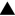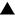Algebra 1 section of MathBitsNotebook.comPolynomialsindicates compatibility to NY Next Generation Math Standards (NGMS)
*
Polynomials (definition, degree, terms, standard form, closure)Polynomials and Algebra Tiles (connection to integers, base 10, base x, algebra tiles)Addition and Subtraction of Polynomials (horizontal & vertical methods)Multiply Monomial by Polynomial (monomial by monomial; monomial by polynomial)Multiply Binomial by Binomial (distributive, vertical, grid, FOIL methods)Special Binomial Products (squaring; product of sum and difference; simple cubing)Multiplication of Polynomials (more combinations of multiplying polynomials)Mindful Algebraic Calculations - PolynomialsPRACTICE: • Polynomial Basics (degree, identify, closure, vocabulary)• Adding and Subtracting Polynomials• Multiplying by a Monomial• Multiplying Binomials• Multiplying Polynomials (combinations of monomials, binomials, trinomials, polynomials)• Visualizing Polynomials (working with geometric figures)• Application Problems with Polynomials (using polynomial expressions)• Dividing by Monomial Practice• Dividing by Binomial Practice